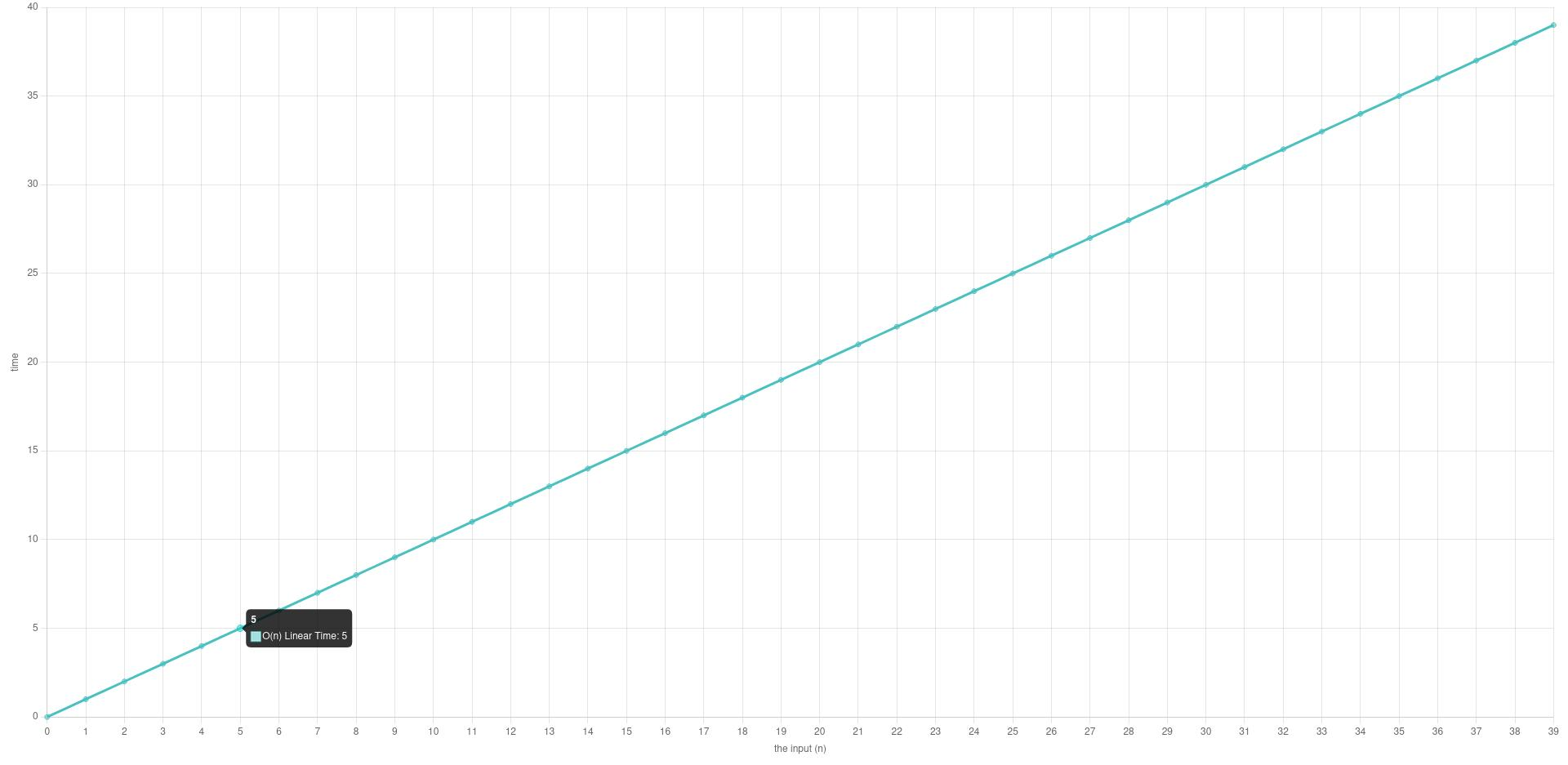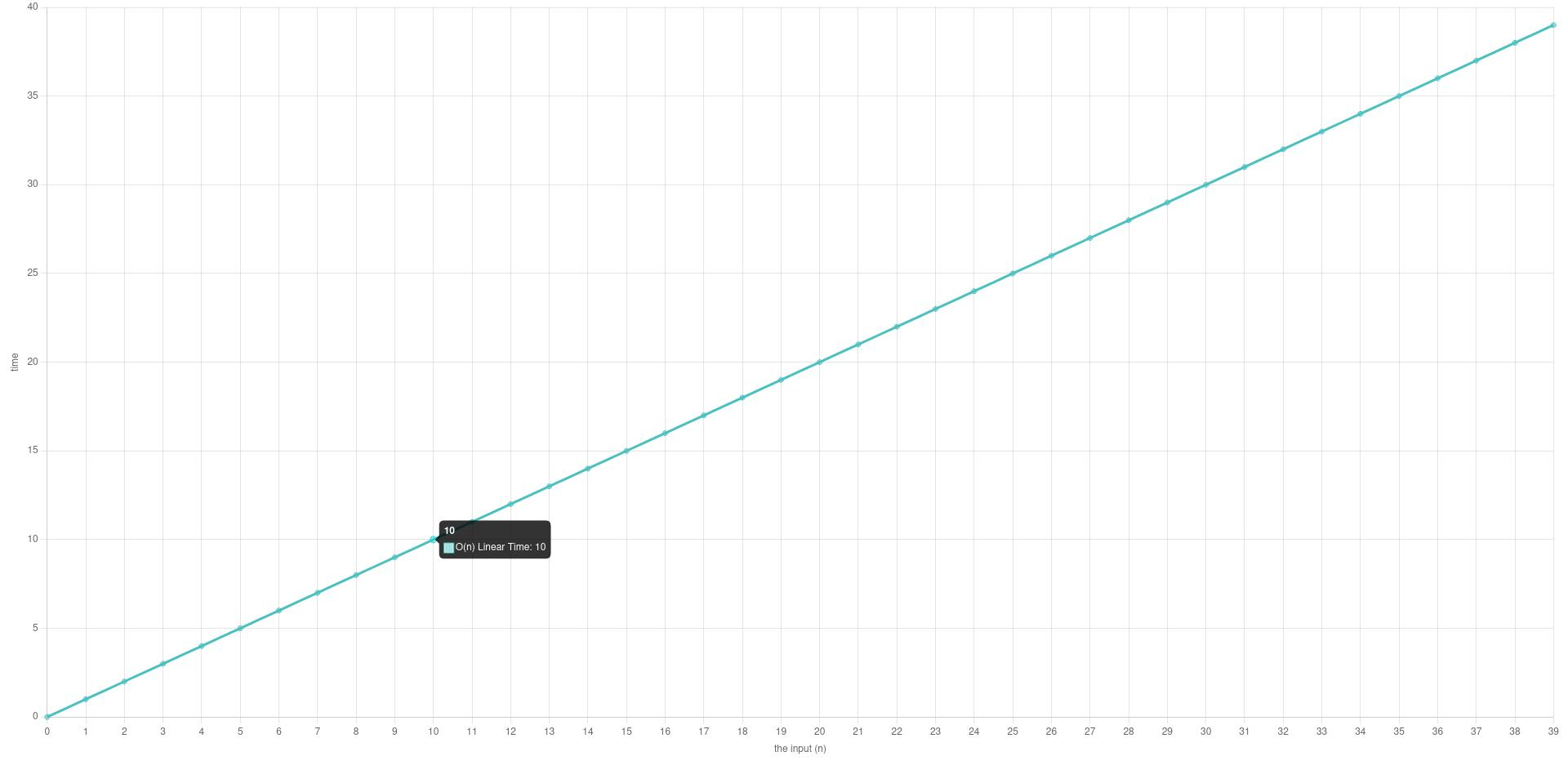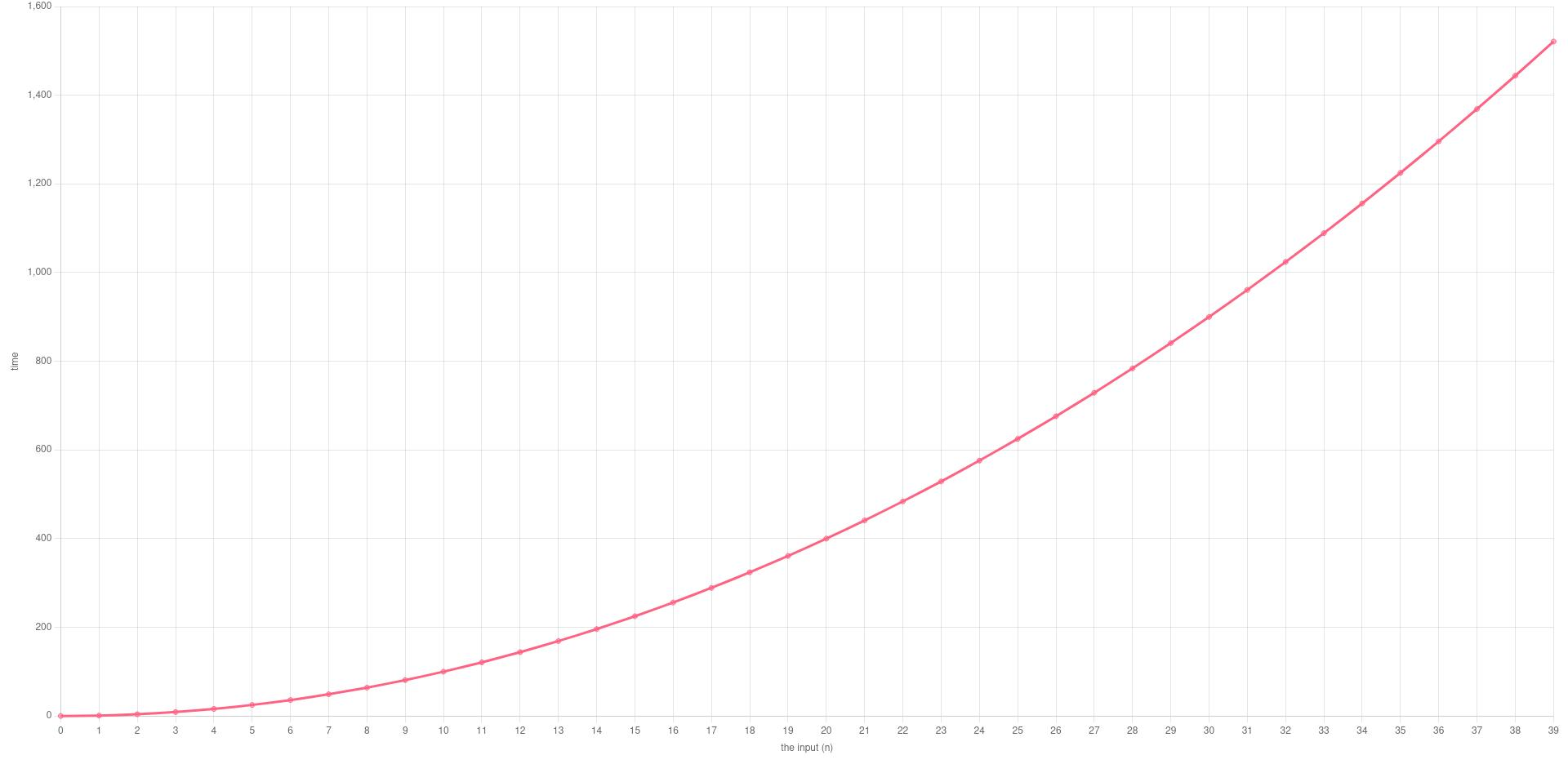# Avoiding Nested Loops to Turn O(N²) into O(N)Avoiding nested loops to turn O(N²) into O(N) time complexity can significantly improve the performance of your programs. One of the most common mistakes made in programming is the use of nested loops, which can lead to performance issues and slow down the execution of a program. Nested loops are used when we need to iterate over a data structure, such as an array or a list, and perform a certain operation on each element. However, nested loops can turn the complexity of our algorithm into O(N²), which can cause our program to slow down or even crash.

In this article, we will discuss the drawbacks of nested loops and how avoiding them can improve programs’ efficiency. So let’s dive in!

FLASH SALES
\$29.95
NEW
\$249.00

### What is Time Complexity?

Time complexity is a measure of the efficiency of an algorithm, representing the amount of time it takes to run as a function of the size of its input. It indicates how the running time of the algorithm grows as the input size increases, and helps to predict how well the algorithm will perform on larger inputs. Time complexity is typically expressed using Big O notation, which provides an upper bound on the growth rate of the running time. Algorithms with lower time complexity are generally more efficient and desirable, as they can handle larger inputs in a reasonable amount of time. To measure your application’s performance you should load-test them. Read this article to learn more about load testing.

### O(N) Time Complexity

O(N) time complexity refers to an algorithm that has a linear relationship between the input size and the time it takes to run. In other words, if the size of the input increases by a factor of N, the running time of the algorithm will also increase by a factor of N.For example, consider a C# program that sums up all the elements in an array of integers:

This program has a time complexity of O(N), where N is the number of elements in the array. This is because the program iterates over each element in the array once, and the time it takes to execute the loop grows linearly in proportion to the size of the array. As the size of the array grows, the time it takes to run the program also grows linearly.

In our example, if we call the `Sum` function with the input size (N) of 5:

The loop iterates 5 times:And if we increase the N (the input size) to 10:

The for loop iterated 10 times.### O(N²) Time Complexity

O(N²) time complexity refers to algorithms that have quadratic relationships between their input size and the time they take to run. In other words, if the size of the input increases by a factor of N, the running time of the algorithm will increase by a factor of N².For example, consider a C# program that compares all pairs of elements in an array of integers:

Let’s call it

The output is:

This program has a time complexity of O(N²), where N is the number of elements in the array. This is because the program iterates over each element in the array twice, resulting in a total of N² iterations. As the size of the array grows, the time it takes to run the program increases quadratically. Therefore, this algorithm is not efficient for large inputs. Did you know that we offer a unique online course that boosts your C# career? Check it out here!

### The Drawbacks of Nested Loops

When we have nested loops, we are essentially iterating over the same set of data multiple times, which can be time-consuming and inefficient. As the size of the data set grows, the time it takes to iterate over the data can become exponentially longer, leading to performance issues. In our example, We passed an array of N elements to the `ComparePairs` function which uses nested loops to compare each element to every other element in the array, because of the nested loop, the algorithm’s complexity was O(N²), which means that the time it takes to execute our program would increase exponentially as the size of the array grows.

Nested loops can also be implemented using recursion, but doign so doesn’t affect the time complexity.

The good news is that there are several techniques we can use to avoid nested loops and reduce the complexity of our algorithms.

### Turning O(N²) time complexity to O(N)

Here’s how we can reduce the time complexity of `ComparePairs` function from O(N²) to O(N):

1. Create a variable to keep track of the minimum element seen so far.
2. Iterate over each element in the array and compare it with the minimum element. If the current element is less than the minimum, update the minimum and print the pair.
3. By the end of the loop, we have found all pairs where the left element is the minimum.

Here’s what the modified code would look like:

This modified algorithm has a time complexity of O(n), where n is the number of elements in the input array. The loop iterates over each element once, and each operation inside the loop takes constant time. Therefore, the total time taken is proportional to the input size, making it much faster than the original algorithm with a time complexity of O(n²).

### Techniques

Here are a few general techniques you can use to reduce your algorithms’ time complexity:

• Hashing: Hashing can be used to reduce O(N²) to O(N) in some cases. For example, if we need to find two numbers in an array that add up to a target value, we can use a hash table to store the values and their indices. Then, we can iterate through the array once and check if the complement of each element exists in the hash table, in O(1) time.
• Memoization: Memoization can be used to reduce O(N²) to O(N) for recursive algorithms by avoiding redundant computations. For example, in the longest common subsequence problem, if we memoize the values of the subsequence that we have already calculated, we can reduce the time complexity from O(2^n) to O(N^2).
• Dynamic programming: Dynamic programming can be used to reduce O(N²) to O(N) by breaking down a problem into smaller subproblems and solving each subproblem only once. For example, the longest increasing subsequence problem can be solved using dynamic programming, where we break down the problem into smaller subproblems and solve them in a bottom-up manner.
• Two pointers: Two pointers can be used to reduce O(N²) to O(N) for searching, sorting, and other problems. For example, in the problem of finding a pair of elements in a sorted array that sum to a given target value, we can use two pointers to iterate over the array in O(N) time.
• Greedy algorithms: Greedy algorithms can be used to reduce O(N²) to O(N) for optimization problems. For example, in the problem of scheduling jobs with deadlines and profits, we can use a greedy algorithm to select the jobs with the highest profits and the earliest deadlines, in O(N log N) time.
• Sorting: Sorting can be used to reduce O(N²) to O(N log N) or O(N) in some cases. For example, the closest pair of points problem can be solved using a divide and conquer algorithm that sorts the points first and then uses a linear algorithm to find the closest pair, in O(N log N) time.

### Conclusion

In conclusion, nested loops can cause performance issues and slow down the execution of our programs. By avoiding nested loops we can reduce the complexity of our algorithms from O(N²) to O(N), or even better. By using techniques introduced in this article, we can avoid nested loops and reduce the complexity of our algorithms. This can significantly improve the performance of our programs and make them more efficient. As a programmer, it’s important to keep these techniques in mind when designing and implementing algorithms. If you want to skyrocket your C# career, check out our powerful ASP.NET full-stack web development course that also covers test-driven development.